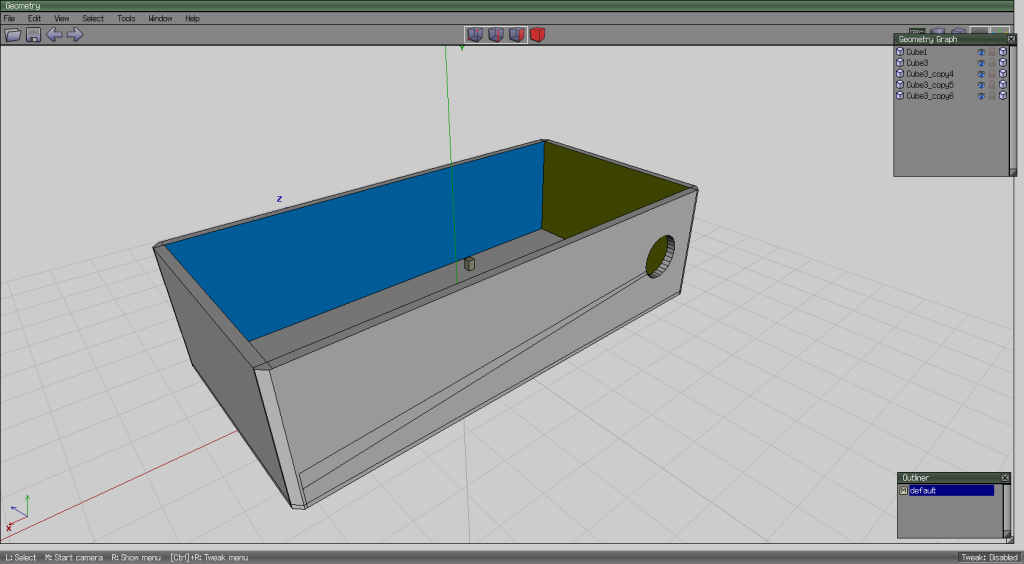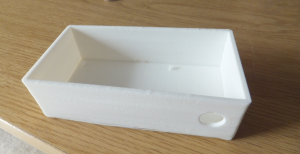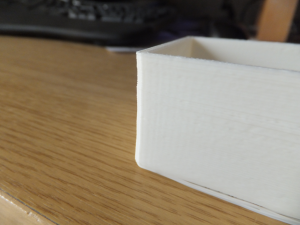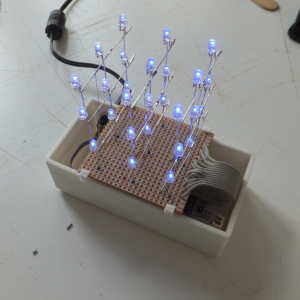## 3D Printed box for the 3x3x3 LED cube

The next thing I thought I’d create was a box for the circuit board of the 3x3x3 LED cube. Seems like it should be easy (famous last words). Note to the people on YouTube who make Wings3D seem so simple and easy and can create a car in 20 minutes – I’m hating you right now.

After a few false starts, I finally managed to produce a box, and I even managed to include a hole in the side for a panel mount socket for powering the cube. I had originally planned to make a lid as well, but since it took me about 3 hours just to make the box, I decided to give the lid a miss.I also included some small pillars on the bottom that the circuit board can rest on. There’s a few extraneous edges in my model that I couldn’t get rid of – still a lot to learn with WIngs3D.

Printing the box took about 3 hours.One thing that was concerning, the slicing program – ReplicatorG/Skeinforge (50) – produced a tool path the shook the printer a great deal. As it was printing the walls of the box, which are about 2mm thick, it did one layer length ways, and then the next layer crossways which creates a stronger bond between the layers. But when doing the printing crossways, because the travel was so short there was a lot of vibration as the print head went back and forth quickly. I think this vibration caused the glass plate on the print bed (which is held in place with some bulldog clips) to shift which meant the walls ended up being a bit wavy:-So I need to work out how to get the slicing program to produce a better tool path. Maybe slow the printhead down when doing short distances – don’t know if that’s possible.

Next thing was how to hold the board with the LEDs on to the box. If I’d thought about it earlier, I could have incorporated these into the box directly. I created some little brackets which fit over the wall of the box, and then hold the LED board.

I printed 4 of these. They only took a couple of minutes each.

Final result.## MVLightning.cpp

```/* Project: 3x3x3 Mono LED Cube (r3).
* File: MVLightning.cpp
* Description:
*     Movie -- mv_Lightning
*         Dark clouds, and occasional flashes of lightning.
*
* Copyright (C) 2014 Marc Symonds
* All rights reserved.
*
* This software may be used and redistribtued, with or without
* modification, as long as it is understood that this software
* is provided as-is without any explicit or implied warranties
* of merchantablity or fitness of purpose.
*/

#include "Arduino.h"
#include "Movies.h"
#include "DisplayFrame.h"

void mv_Lightning()
{
unsigned long ts, m;
unsigned long tl, tf;
byte i, p, y, z, mode, cnt;
Light cloud;
Point l1, l2;

y = 0;
z = 0;
for (i = 0 ; i < 9 ; i++)
{
p = POS(2, y, z);

displayFrame[p] = random(1, 3);

cloud[i].up = (displayFrame[p] < 2);
cloud[i].t = millis() + random(250, 750);

if (++y > 2)
{
y = 0;
++z;
}
}

ts = millis() + (DEF_MOVIE_TIME * 3);

tl = millis() + random(2000, 5000);
mode = 0;

y = 0;
z = 0;
i = 0;
m = millis();
while (m < ts)
{
if (m > cloud[i].t)
{
p = POS(2, y, z);
displayFrame[p] += (cloud[i].up ? 1 : -1);
if (displayFrame[p] < 2)
cloud[i].up = true;
else if (displayFrame[p] > 1)
cloud[i].up = false;

cloud[i].t = m + random(250, 750);
}

if (mode > 0)
{
if (m > tf)
{
if (mode == 1)
{
displayFrame[l1.Pos()] = 7;
displayFrame[l2.Pos()] = 7;
mode = 2;
}
else
{
displayFrame[l1.Pos()] = 0;
displayFrame[l2.Pos()] = 0;

if (--cnt < 1)
{
mode = 0;
tl = millis() + random(2000, 5000);
}
else
mode = 1;
}

tf = m + 30;
}
}
else if (m > tl)
{
l1.x = 1;
l1.y = random(0, 3);
l1.z = random(0, 3);

l2.x = 0;
do
{
l2.y = l1.y + random(0, 3) - 1;
} while (l2.y > 2);

do
{
l2.z = l1.z + random(0, 3) - 1;
} while (l2.z > 2);

mode = 1;
cnt = random(5, 8);

tf = m;
}

if (++y > 2)
{
y = 0;
if (++z > 2)
z = 0;
}

if (++i > 8)
i = 0;

m = millis();
}

}
```

## MVBoxy.cpp

```/* Project: 3x3x3 Mono LED Cube (r3).
* File: MVBoxy.cpp
* Description:
*     Movie -- mv_Boxy
*         Randonly display a growing and shrinking box.
*         (as well as you can do in 3x3x3).
*
* Copyright (C) 2014 Marc Symonds
* All rights reserved.
*
* This software may be used and redistributed, with or without
* modification, as long as it is understood that this software
* is provided as-is without any explicit or implied warranties
* of merchantablity or fitness of purpose.
*/

#include "Arduino.h"
#include "Movies.h"
#include "DisplayFrame.h"

void mv_Boxy()
{
unsigned long ts;
unsigned long tbox = 1; // 0xB000000000000000000000000001;
unsigned long sbox = 13851; //0XB000000000000011011000011011;
unsigned long lbox = 129948655; //0XB111101111101101101111101111;
int spd;
POSTransFPtr trans;

trans = GetPOSTrans(random(0, 8));

ts = millis() + (DEF_MOVIE_TIME / 2);

while (millis() < ts)
{
spd = random(50, 90);

df_DisplayMonoFrame(trans, tbox);
delay(spd);
df_DisplayMonoFrame(trans, sbox);
delay(spd);
df_DisplayMonoFrame(trans, lbox);
delay(spd * 4);

trans = GetPOSTrans(random(0, 8));
df_DisplayMonoFrame(trans, sbox);
delay(spd);
df_DisplayMonoFrame(trans, tbox);
delay(spd);
df_clear();

delay(spd);
}
}
```

## Movies.h

```/* Project: 3x3x3 Mono LED Cube (r3).
* File: Movies.h
* Description: Definitions for movie functions.
*
* Copyright (C) 2014 Marc Symonds
* All rights reserved.
*
* This software may be used and redistributed, with or without
* modification, as long as it is understood that this software
* is provided as-is without any explicit or implied warranties
* of merchantablity or fitness of purpose.
*/

#ifndef Movies_h
#define Movies_h

#define DEF_MOVIE_TIME 10000

void mv_TwinkleAll();
void mv_Glitter();
void mv_Pulse();
void mv_Jitter();
void mv_EdgeChase();
void mv_EdgeChase2();
void mv_Rain();
void mv_AllOn();
void mv_Flashy();
void mv_EdgeTumble();
void mv_Counter();
void mv_Snake();
void mv_Boxy();
void mv_Lightning();
#endif
```

## MVTwinkleAll.cpp

```/* Project: 3x3x3 Mono LED Cube (r3).
* File: MVTwinkleAll.cpp
* Description:
*     Movie -- mv_TwinkleAll
*         All LEDs are randomly faded in an out.
*         Similar to mv_Flashy, but with a subtle difference.
*
* Copyright (C) 2014 Marc Symonds
* All rights reserved.
*
* This software may be used and redistributed, with or without
* modification, as long as it is understood that this software
* is provided as-is without any explicit or implied warranties
* of merchantablity or fitness of purpose.
*/

#include "Arduino.h"
#include "Movies.h"
#include "DisplayFrame.h"

void mv_TwinkleAll()
{
unsigned long ts;
byte x;
byte m;

ts = millis() + DEF_MOVIE_TIME;

for (x = 0 ; x < 27 ; x++)
m[x] = (displayFrame[x] == MAXBRIGHTNESSLEVEL);

while (millis() < ts)
{
for (x = 0 ; x < 27 ; x++)
{
if (m[x])
{
if (random(10) > 6)
{
if (--displayFrame[x] == 0)
m[x] = false;
}
}
else
{
if (random(10) > 4)
{
if (++displayFrame[x] >= MAXBRIGHTNESSLEVEL)
m[x] = true;
}
}
}

delay(50);
}

}
```

## MVSnake.cpp

```/* Project: 3x3x3 Mono LED Cube (r3).
* File: MVSnake.cpp
* Description:
*     Movie -- mv_Snake
*
* Copyright (C) 2014 Marc Symonds
* All rights reserved.
*
* This software may be used and redistributed, with or without
* modification, as long as it is understood that this software
* is provided as-is without any explicit or implied warranties
* of merchantablity or fitness of purpose.
*/

#include "Arduino.h"
#include "Movies.h"
#include "DisplayFrame.h"

#define SNAKELEN 4

void mv_Snake()
{
unsigned long ts, t;
int ss, sl, sp, i, j, k;
byte nx, ny, nz;

ts = millis() + DEF_MOVIE_TIME;

points = Point((byte)random(0, 3), (byte)random(0, 3), (byte)random(0, 3));
sl = 1;
ss = 0;
displayFrame[points.Pos()] = MAXBRIGHTNESSLEVEL;

sp = random(100, 500);

while (millis() < ts)
{
t = millis() + sp;

do
{
nx = points[ss].x;
ny = points[ss].y;
nz = points[ss].z;

switch(random(0, 3))
{
case 0:
nx = nx + random(0, 3) - 1;
break;

case 1:
ny = ny + random(0, 3) - 1;
break;

default:
nz = nz + random(0, 3) - 1;
break;
}
} while (millis() < t && (nx > 2 || ny > 2 || nz > 2 || displayFrame[POS(nx, ny, nz)] != 0));

while (millis() < t)
;

if (nx > 2 || ny > 2 || nz > 2 || displayFrame[POS(nx, ny, nz)] != 0)
{
for (i = 0 ; i < 27 ; i++)
displayFrame[i] = MAXBRIGHTNESSLEVEL;

delay(50);

df_clear();

points = Point((byte)random(0, 3), (byte)random(0, 3), (byte)random(0, 3));
sl = 1;
ss = 0;
displayFrame[points[ss].Pos()] = MAXBRIGHTNESSLEVEL;
}
else
{
i = ss;
for (j = 0 ; j < sl ; j++)
{
k = points[i].Pos();
if (displayFrame[k] > 0)
--displayFrame[k];
if (displayFrame[k] > 0)
--displayFrame[k];

if (i == 0)
i = SNAKELEN - 1;
else
--i;
}

if (++ss >= SNAKELEN)
ss = 0;

points[ss].Set(nx, ny, nz);
displayFrame[points[ss].Pos()] = MAXBRIGHTNESSLEVEL;

if (sl < SNAKELEN)
++sl;
}
}

df_clear();
}
```

## MVRain.cpp

```/* Project: 3x3x3 Mono LED Cube (r3).
* File: MVRain.cpp
* Description:
*     Movie -- mv_Rain
*         A number of LEDs are lit on the top plane, and they
*         then "fall down" the cude.
*
* Copyright (C) 2014 Marc Symonds
* All rights reserved.
*
* This software may be used and redistributed, with or without
* modification, as long as it is understood that this software
* is provided as-is without any explicit or implied warranties
* of merchantablity or fitness of purpose.
*/

#include "Arduino.h"
#include "Movies.h"
#include "DisplayFrame.h"

class Drop
{
public:
byte pos;
byte count;
unsigned long time;
byte spd;

void reset()
{
pos = POS(2, random(0, 3), random(0, 3));
count = 2;
spd = random(30, 100);
time = millis() + spd;
}

void displayDrop(boolean state)
{
displayFrame[pos] = state ? MAXBRIGHTNESSLEVEL : 0;
}

void drop()
{
if (millis() > time)
{
if (spd == 0)
{
reset();
displayDrop(true);
}
else
{
displayDrop(false);
if (count == 0)
{
spd = 0;
time = millis() + random(50, 100);
}
else
{
--count;
pos -= 3;
displayDrop(true);
time = millis() + spd;
}
}
}
}
};

void mv_Rain()
{
unsigned long ts;
byte y;
Drop drops;

ts = millis() + (DEF_MOVIE_TIME * 2);

for (y = 0 ; y < 2 ; y++)
{
drops[y].reset();
drops[y].displayDrop(true);
}

while (millis() < ts)
{
for (y = 0 ; y < 2 ; y++)
{
drops[y].drop();
}
}

df_clear();
}
```

## MVPulse.cpp

```/* Project: 3x3x3 Mono LED Cube (r3).
* File: MVPulse.cpp
* Description:
*     Movie -- mv_Pulse
*         Fade in and out all LEDs together.
*
* Copyright (C) 2014 Marc Symonds
* All rights reserved.
*
* This software may be used and redistributed, with or without
* modification, as long as it is understood that this software
* is provided as-is without any explicit or implied warranties
* of merchantablity or fitness of purpose.
*/

#include "Arduino.h"
#include "Movies.h"
#include "DisplayFrame.h"

void mv_Pulse()
{
unsigned long ts;
byte x, y;

ts = millis() + (DEF_MOVIE_TIME / 2);

while (millis() < ts)
{
for (y = 0 ; y <= MAXBRIGHTNESSLEVEL ; y++)
{
for (x = 0 ; x < 27 ; x++)
displayFrame[x] = y;

delay(50);
}

delay(100);

for (y = MAXBRIGHTNESSLEVEL - 1 ; y > 0 ; y--)
{
for (x = 0 ; x < 27 ; x++)
displayFrame[x] = y;

delay(50);
}

df_clear();

delay(500);
}
}
```

## MVJitter.cpp

```/* Project: 3x3x3 Mono LED Cube (r3).
* File: MVJitter.cpp
* Description:
*     Movie -- mv_Jitter
*         A single LED moves quickly and randomly around the
*         cube.
*
* Copyright (C) 2014 Marc Symonds
* All rights reserved.
*
* This software may be used and redistributed, with or without
* modification, as long as it is understood that this software
* is provided as-is without any explicit or implied warranties
* of merchantablity or fitness of purpose.
*/

#include "Arduino.h"
#include "Movies.h"
#include "DisplayFrame.h"

void mv_Jitter()
{
unsigned long ts, t;
byte x, y, z, nx, ny, nz;

ts = millis() + DEF_MOVIE_TIME;

x = random(0, 3);
y = random(0, 3);
z = random(0, 3);
nx = x;
ny = y;
nz = z;

displayFrame[POS(x, y, z)] = MAXBRIGHTNESSLEVEL;

while (millis() < ts)
{
t = millis() + 80;

do
{
// Work out the next LED to light, adjacent to the current LED.

switch(random(0, 3))
{
case 0:
switch(random(0, 3))
{
case 0:
if (nx > 0)
--nx;
break;
case 2:
if (nx < 2)
++nx;
break;
}
break;

case 1:
switch(random(0, 3))
{
case 0:
if (ny > 0)
--ny;
break;
case 2:
if (ny < 2)
++ny;
break;
}
break;

default:
switch(random(0, 3))
{
case 0:
if (nz > 0)
--nz;
break;
case 2:
if (nz < 2)
++nz;
break;
}
break;
}
} while ( x == nx && y == ny && z == nz);

while (millis() < t)
;

displayFrame[POS(x,y,z)] = 0;
displayFrame[POS(nx,ny,nz)] = MAXBRIGHTNESSLEVEL;

x = nx;
y = ny;
z = nz;
}

displayFrame[POS(nx,ny,nz)] = 0;
}
```

## MVGlitter.cpp

```/* Project: 3x3x3 Mono LED Cube (r3).
* File: MVGlitter.cpp
* Description:
*     Movie -- mv_Glitter
*         Lights random LEDs for short periods of time creating
*         a "glitter" effect.
*
* Copyright (C) 2014 Marc Symonds
* All rights reserved.
*
* This software may be used and redistribtued, with or without
* modification, as long as it is understood that this software
* is provided as-is without any explicit or implied warranties
* of merchantablity or fitness of purpose.
*/

#include "Arduino.h"
#include "Movies.h"
#include "DisplayFrame.h"

void mv_Glitter()
{
unsigned long ts;
byte x;
byte m;
byte n = {0, 0, 0};

ts = millis() + DEF_MOVIE_TIME;

while (millis() < ts)
{
for (x = 0 ; x < 3 ; x++)
{
if (n[x] == 0)
{
displayFrame[m[x]] = 0;
m[x] = random(0, 27);
n[x] = random(30, 50);
displayFrame[m[x]] = MAXBRIGHTNESSLEVEL;
}
else
--n[x];
}
delay(1);
}

for (x = 0 ; x < 3 ; x++)
displayFrame[m[x]] = 0;
}
```